Topics: AI | Data Science | Data Visualization | Deep Learning | Machine Learning | NLP | Python | R | Statistics

KDnuggets Home » News » 2020 » Apr » Tutorials, Overviews » Data Transformation: Standardization vs Normalization ( 20:n17 )

# Data Transformation: Standardization vs Normalization

Increasing accuracy in your models is often obtained through the first steps of data transformations. This guide explains the difference between the key feature scaling methods of standardization and normalization, and demonstrates when and how to apply each approach.Data transformation is one of the fundamental steps in the part of data processing. When I first learnt the technique of feature scaling, the terms scalestandardise, and normalise are often being used. However, it was pretty hard to find information about which of them I should use and also when to use. Therefore, I’m going to explain the following key aspects in this article:

• the difference between Standardisation and Normalisation
• when to use Standardisation and when to use Normalisation
• how to apply feature scaling in Python

### What does Feature Scaling mean?

In practice, we often encounter different types of variables in the same dataset. A significant issue is that the range of the variables may differ a lot. Using the original scale may put more weights on the variables with a large range. In order to deal with this problem, we need to apply the technique of features rescaling to independent variables or features of data in the step of data pre-processing. The terms normalisation and standardisation are sometimes used interchangeably, but they usually refer to different things.

The goal of applying Feature Scaling is to make sure features are on almost the same scale so that each feature is equally important and make it easier to process by most ML algorithms.

### Example

This is a dataset that contains an independent variable (Purchased) and 3 dependent variables (Country, Age, and Salary). We can easily notice that the variables are not on the same scale because the range of Age is from 27 to 50, while the range of Salary going from 48 K to 83 K. The range of Salary is much wider than the range of Age. This will cause some issues in our models since a lot of machine learning models such as k-means clustering and nearest neighbour classification are based on the Euclidean Distance.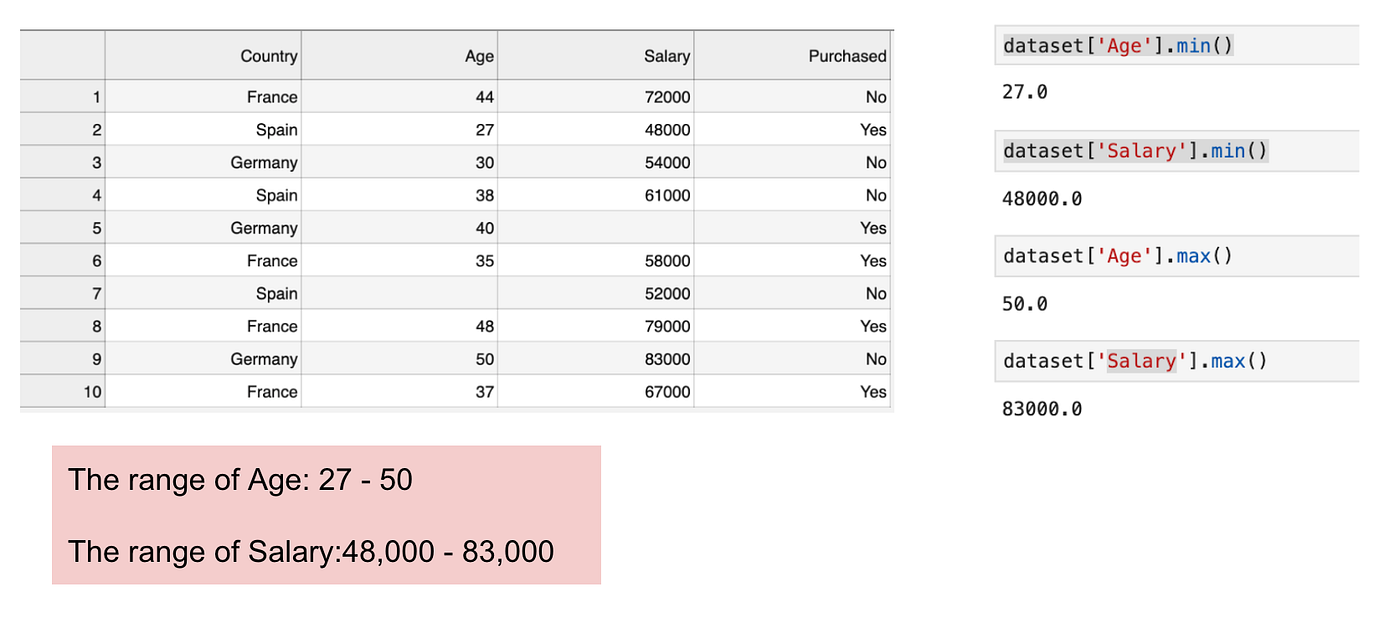Focusing on age and salary.

When we calculate the equation of Euclidean distance, the number of (x2-x1)² is much bigger than the number of (y2-y1)² which means the Euclidean distance will be dominated by the salary if we do not apply feature scaling. The difference in Age contributes less to the overall difference. Therefore, we should use Feature Scaling to bring all values to the same magnitudes and, thus, solve this issue. To do this, there are primarily two methods called Standardisation and Normalisation.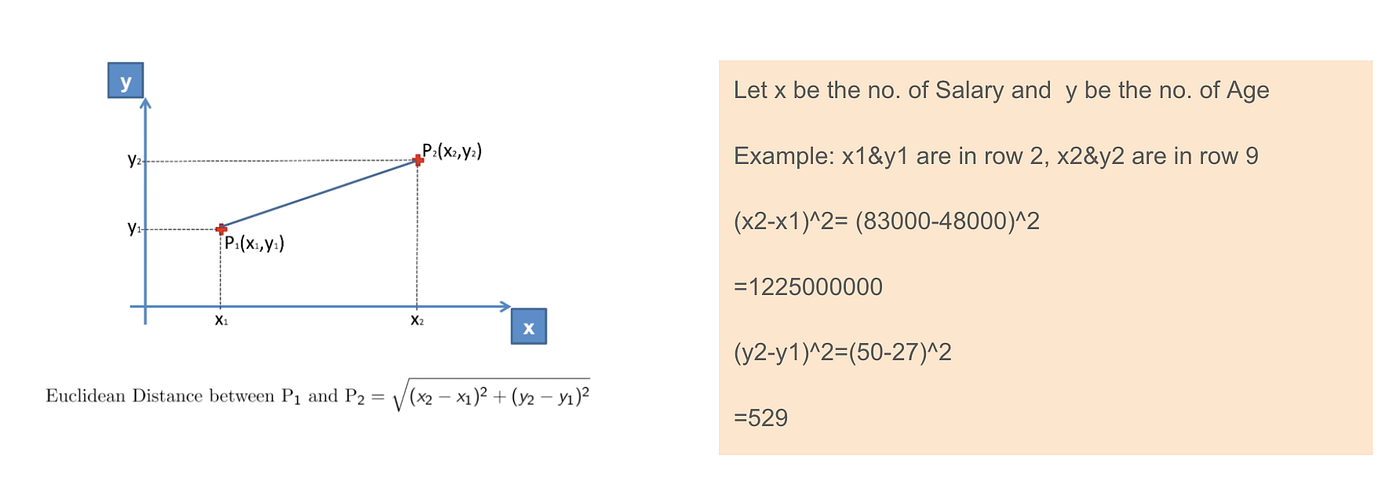Euclidean distance application.

### Standardisation

The result of standardization (or Z-score normalization) is that the features will be rescaled to ensure the mean and the standard deviation to be 0 and 1, respectively. The equation is shown below: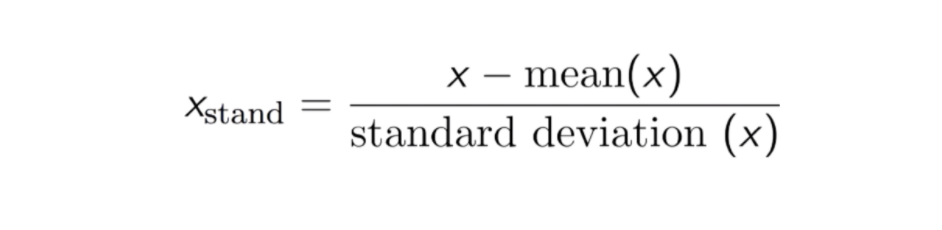This technique is to re-scale features value with the distribution value between 0 and 1 is useful for the optimization algorithms, such as gradient descent, that are used within machine learning algorithms that weight inputs (e.g., regression and neural networks). Rescaling is also used for algorithms that use distance measurements, for example, K-Nearest-Neighbours (KNN).

Code

```#Import library
from sklearn.preprocessing import StandardScaler
sc_X = StandardScaler()
sc_X = sc_X.fit_transform(df)
#Convert to table format - StandardScaler
sc_X = pd.DataFrame(data=sc_X, columns=["Age", "Salary","Purchased","Country_France","Country_Germany", "Country_spain"])
sc_X

```

### Max-Min Normalization

Another common approach is the so-called Max-Min Normalization (Min-Max scaling). This technique is to re-scales features with a distribution value between 0 and 1. For every feature, the minimum value of that feature gets transformed into 0, and the maximum value gets transformed into 1. The general equation is shown below:The equation of Max-Min Normalization.

Code

```from sklearn.preprocessing import MinMaxScaler
scaler = MinMaxScaler()
scaler.fit(df)
scaled_features = scaler.transform(df)
#Convert to table format - MinMaxScaler
df_MinMax = pd.DataFrame(data=scaled_features, columns=["Age", "Salary","Purchased","Country_France","Country_Germany", "Country_spain"])

```

### Standardisation vs Max-Min Normalization

In contrast to standardisation, we will obtain smaller standard deviations through the process of Max-Min Normalisation. Let me illustrate more in this area using the above dataset.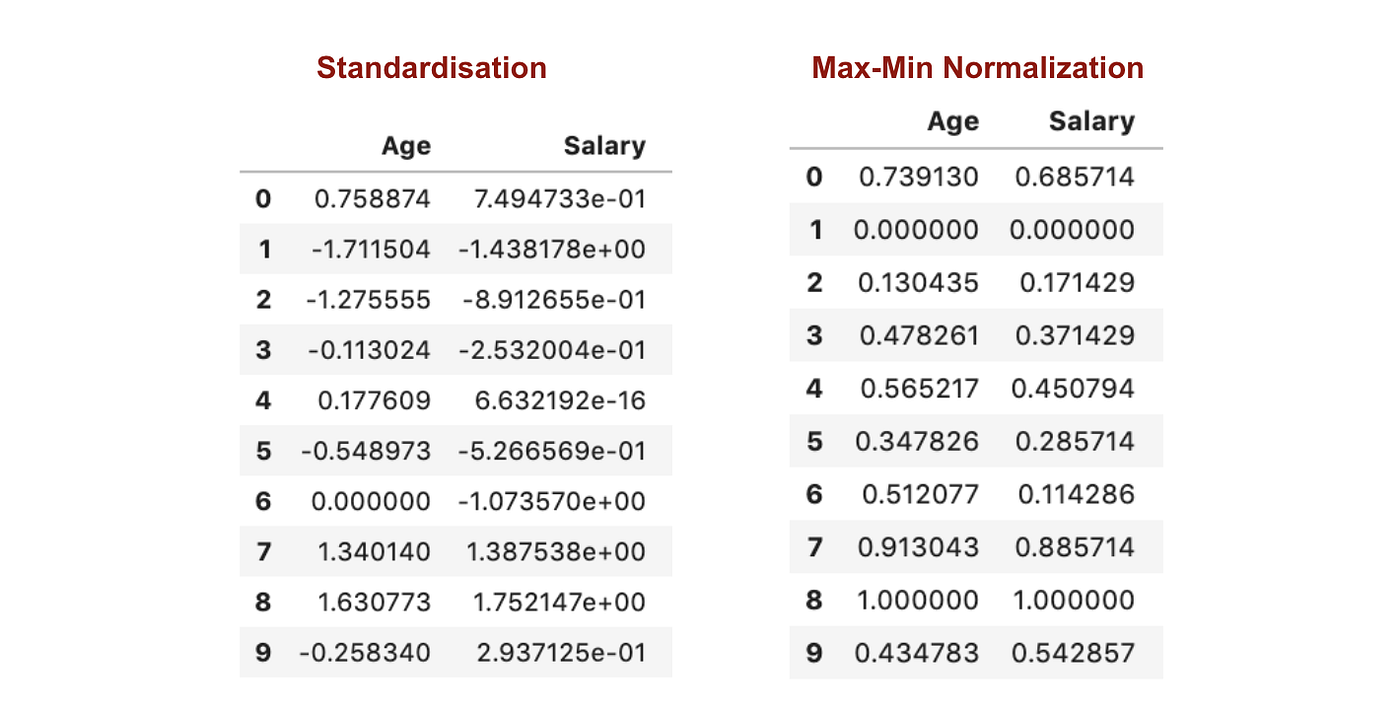After Feature scaling.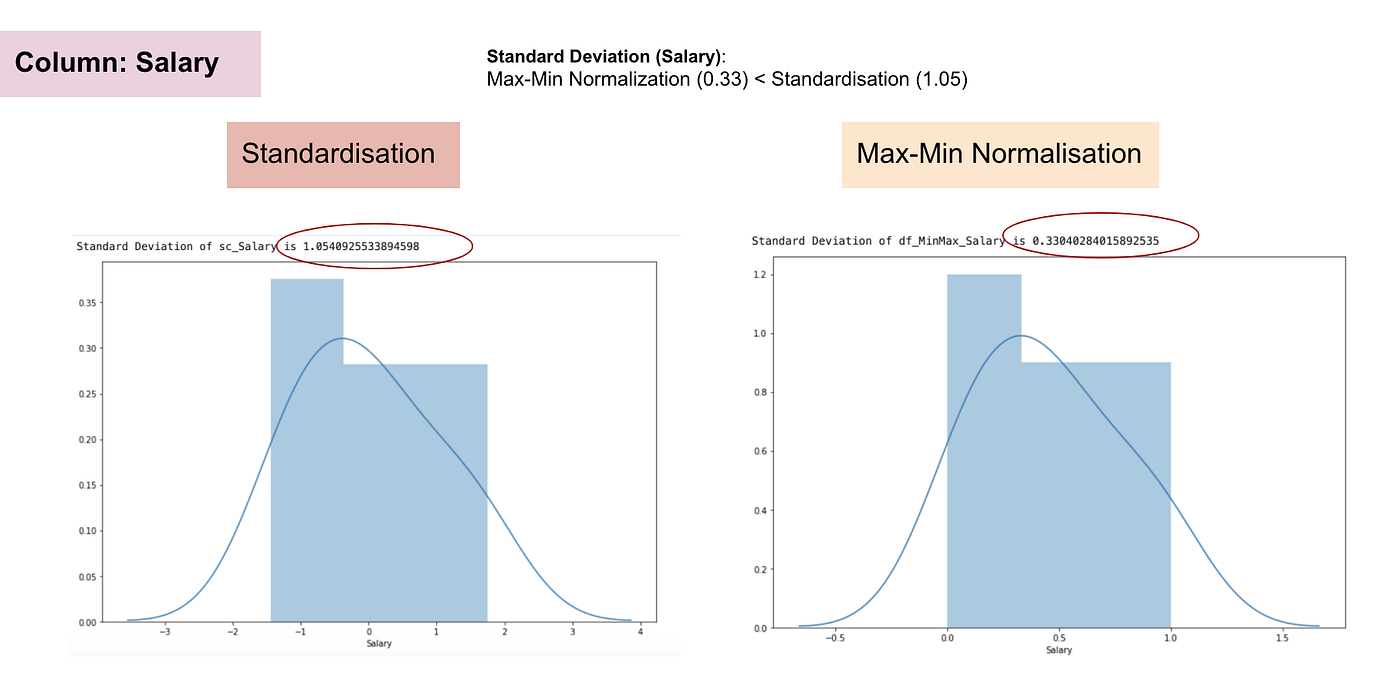Normal distribution and Standard Deviation of Salary.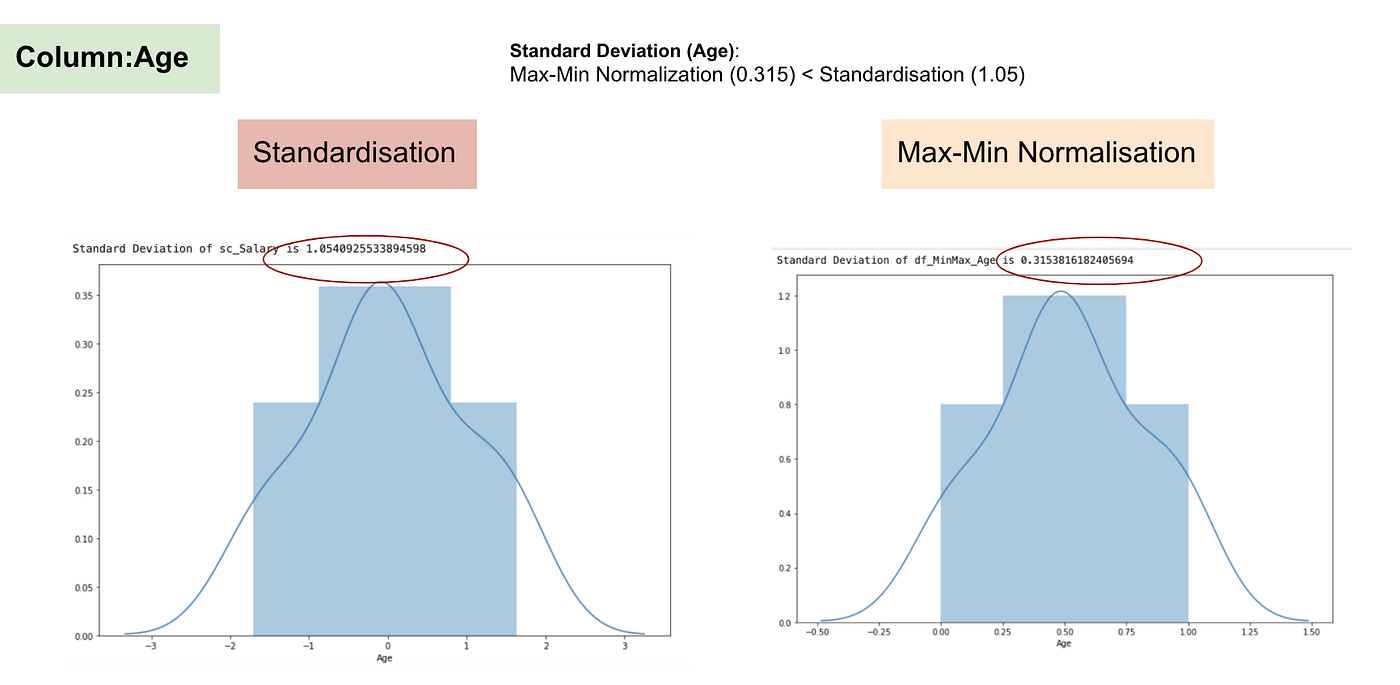Normal distribution and Standard Deviation of Age.

From the above graphs, we can clearly notice that applying Max-Min Nomaralisation in our dataset has generated smaller standard deviations (Salary and Age) than using Standardisation method. It implies the data are more concentrated around the mean if we scale data using Max-Min Nomaralisation.

As a result, if you have outliers in your feature (column), normalizing your data will scale most of the data to a small interval, which means all features will have the same scale but does not handle outliers well. Standardisation is more robust to outliers, and in many cases, it is preferable over Max-Min Normalisation.

### When Feature Scaling matters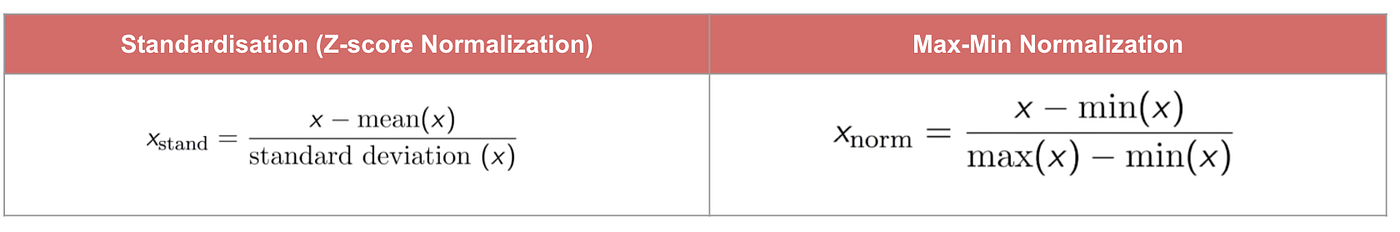Some machine learning models are fundamentally based on distance matrix, also known as the distance-based classifier, for example, K-Nearest-Neighbours, SVM, and Neural Network. Feature scaling is extremely essential to those models, especially when the range of the features is very different. Otherwise, features with a large range will have a large influence in computing the distance.

Max-Min Normalisation typically allows us to transform the data with varying scales so that no specific dimension will dominate the statistics, and it does not require making a very strong assumption about the distribution of the data, such as k-nearest neighbours and artificial neural networks. However, Normalisation does not treat outliners very well. On the contrary, standardisation allows users to better handle the outliers and facilitate convergence for some computational algorithms like gradient descent. Therefore, we usually prefer standardisation over Min-Max Normalisation.

Example: What algorithms need feature scaling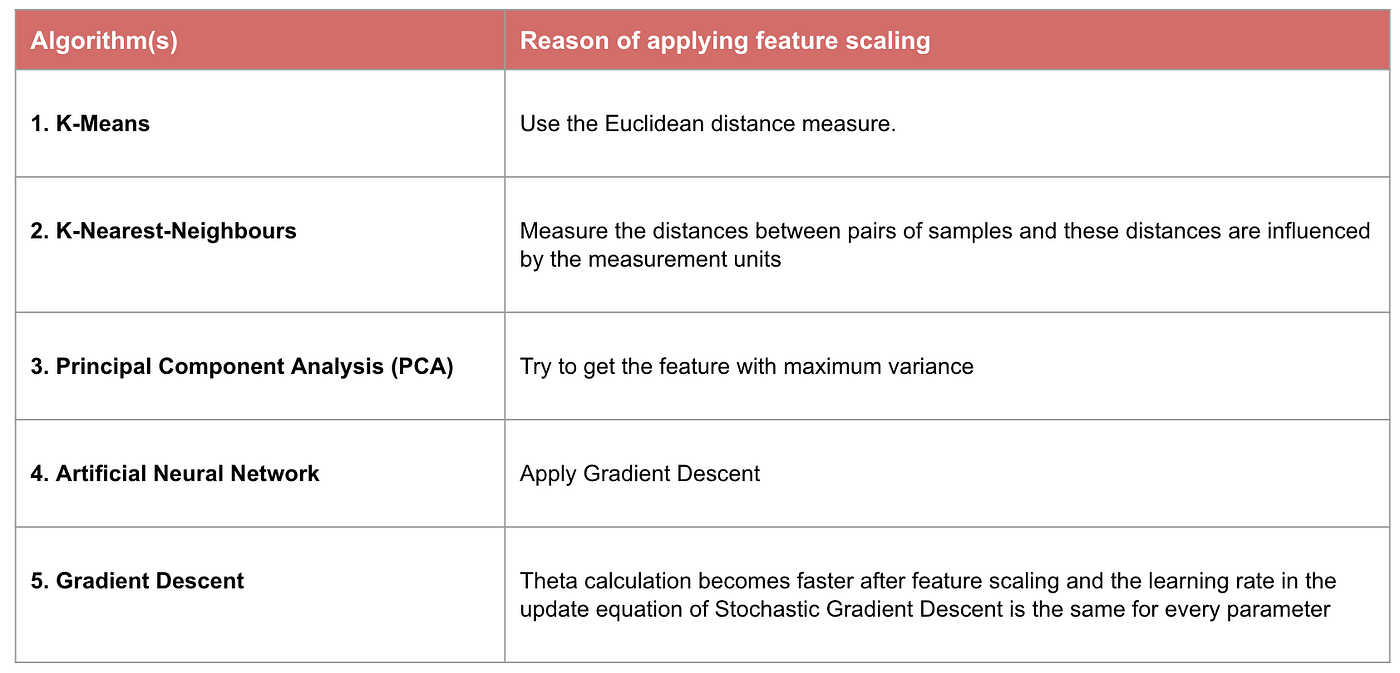Note: If an algorithm is not distance-based, feature scaling is unimportant, including Naive Bayes, Linear Discriminant Analysis, and Tree-Based models (gradient boosting, random forest, etc.).

### Summary: Now you should know

• the objective of using Feature Scaling
• the difference between Standardisation and Normalisation
• the algorithms that need to apply Standardisation or Normalisation
• applying feature scaling in Python

Please find the code and dataset here.

Original. Reposted with permission.

Related:

Top Stories Past 30 Days
Most Popular
Most SharedGet KDnuggets, a leading newsletter on AI, Data Science, and Machine Learning